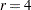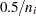Cautions

Effective Sample Size

Since the method depends on asymptotic approximations, you need to be careful that the sample sizes are sufficiently large to support the asymptotic normal distributions of the response functions. A general guideline is that you would like to have an effective sample size of at least 25 to 30 for each response function that is being analyzed. For example, if you have one dependent variable andresponse levels, and you use the standard response functions to compute three generalized logits for each population, then you would like the sample size of each population to be at least 75. Moreover, the subjects should be dispersed throughout the table so that less than 20 percent of the response functions have an effective sample size less than 5. For example, if each population had less than 5 subjects in the first response category, then it would be wiser to pool this category with another category rather than to assume the asymptotic normality of the first response function. Or, if the dependent variable is ordinally scaled, an alternative is to request the mean score response function rather than three generalized logits.

If there is more than one dependent variable, and you specify RESPONSE MEANS, then the effective sample size for each response function is the same as the actual sample size. Thus, a sample size of 30 could be sufficient to support four response functions, provided that the functions are the means of four dependent variables.

A Singular Covariance Matrix

If there is a singular (noninvertible) covariance matrix for the response functions in any population, then PROC CATMOD writes an error message and stops processing. You have several options available to correct this problem:

• You can reduce the number of response functions according to how many can be supported by the populations with the smallest sample sizes.

• If there are three or more levels for any independent variable, you can pool the levels into a fewer number of categories, thereby reducing the number of populations. However, your interpretation of results must be done more cautiously since such pooling implies a different sampling scheme and masks any differences that existed among the pooled categories.

• If there are two or more independent variables, you can delete at least one of them from the model. However, this is just another form of pooling, and the same cautions that apply to the previous option also apply here.

• If there is one independent variable, then, in some situations, you might simply eliminate the populations that are causing the covariance matrices to be singular.

• You can use the ADDCELL= option in the MODEL statement to add a small amount (for example, 0.5) to every cell frequency, but this can seriously bias the results if the cell frequencies are small.

Zero Frequencies

There are two types of zero cells in a contingency table: structural and sampling. A structural zero cell has an expected value of zero, while a sampling zero cell can have nonzero expected value and can be estimable.

If you use the standard response functions and there are zero frequencies, you should use maximum likelihood estimation (the default is ML=NR) rather than weighted least squares to analyze the data. For weighted least squares analysis, the CATMOD procedure always computes the observed response functions and might need to take the logarithm of a zero proportion. In this case, PROC CATMOD issues a warning and then takes the log of a small value (for the probability) in order to continue, but this can produce invalid results if the cells contain too few observations. Maximum likelihood analysis, on the other hand, does not require computation of the observed response functions and therefore yields valid results for the parameter estimates and all of the predicted values.

For a log-linear model analysis with WLS or ML=NR, PROC CATMOD creates response profiles only for the observed profiles. For any log-linear model analysis with one population (the usual case), the contingency table does not contain zeros, which means that all zero frequencies are treated as structural zeros. If there is more than one population, then a zero in the body of the contingency table is treated as a sampling zero (as long as some population has a nonzero count for that profile). If you fit the log-linear model by using ML=IPF, the contingency table is incomplete and the zeros are treated like structural zeros. If you want zero frequencies that PROC CATMOD would normally treat as structural zeros to be interpreted as sampling zeros, you can specify the ZERO=SAMPLING and MISSING=SAMPLING options in the MODEL statement. Alternatively, you can specify ZERO=1E–20 and MISSING=1E–20.

See Bishop, Fienberg, and Holland (1975) for a discussion of the issues and Example 29.5 for an illustration of a log-linear model analysis of data that contain both structural and sampling zeros.

If you perform a weighted least squares analysis on a contingency table that contains zero cell frequencies, then avoid using the LOG transformation as the first transformation on the observed proportions. In general, it is better to change the response functions or to pool some of the response categories than to settle for the 0.5 correction or to use the ADDCELL= option.

Testing the Wrong Hypothesis

If you use the keyword _RESPONSE_ in the MODEL statement, and you specify MARGINALS, LOGITS, ALOGITS, or CLOGITS in your RESPONSE statement, you might receive the following warning message:

```   Warning: The _RESPONSE_ effect may be testing the wrong
hypothesis since the marginal levels of the
dependent variables do not coincide. Consult the
response profiles and the CATMOD documentation.
```

The following examples illustrate situations in which the _RESPONSE_ effect tests the wrong hypothesis.

Zeros in the Marginal Frequencies

Suppose you specify the following statements:

```data A1;
input Time1 Time2 @@;
datalines;
1 2    2 3    1 3
;
```
```proc catmod;
response marginals;
model Time1*Time2=_response_;
repeated Time 2 / _response_=Time;
run;
```

One marginal probability is computed for each dependent variable, resulting in two response functions. The model is a saturated one: one degree of freedom for the intercept and one for the main effect of Time. Except for the warning message, PROC CATMOD produces an analysis with no apparent errors, but the "Response Profiles" table displayed by PROC CATMOD is as follows:

Response Profiles

Response

Time1

Time2

1

1

2

2

1

3

3

2

3

Since RESPONSE MARGINALS yields marginal probabilities for every level but the last, the two response functions being analyzed are Prob(Time1=1) and Prob(Time2=2). The Time effect is testing the hypothesis that Prob(Time1=1)=Prob(Time2=2). What it should be testing is the hypothesis that

```   Prob(Time1=1) = Prob(Time2=1)
Prob(Time1=2) = Prob(Time2=2)
Prob(Time1=3) = Prob(Time2=3)
```

but there are not enough data to support the test (assuming that none of the probabilities are structural zeros by the design of the study).

The ORDER=DATA Option

Suppose you specify the following statements:

```data a1;
input Time1 Time2 @@;
datalines;
2 1    2 2    1 1    1 2    2 1
;
```
```proc catmod order=data;
response marginals;
model Time1*Time2=_response_;
repeated Time 2 / _response_=Time;
run;
```

As in the preceding example, one marginal probability is computed for each dependent variable, resulting in two response functions. The model is also the same: one degree of freedom for the intercept and one for the main effect of Time. PROC CATMOD issues the warning message and displays the following "Response Profiles" table:

Response Profiles

Response

Time1

Time2

1

2

1

2

2

2

3

1

1

4

1

2

Although the marginal levels are the same for the two dependent variables, they are not in the same order because the ORDER=DATA option specified that they be ordered according to their appearance in the input stream. Since RESPONSE MARGINALS yields marginal probabilities for every level except the last, the two response functions being analyzed are Prob(Time1=2) and Prob(Time2=1). The Time effect is testing the hypothesis that Prob(Time1=2)=Prob(Time2=1). What it should be testing is the hypothesis that

```   Prob(Time1=1) = Prob(Time2=1)
Prob(Time1=2) = Prob(Time2=2)
```

Whenever the warning message appears, look at the "Response Profiles" table or the "One-Way Frequencies" table to determine what hypothesis is actually being tested. For the latter example, a correct analysis can be obtained by deleting the ORDER=DATA option or by reordering the data so that the (1,1) observation is first.